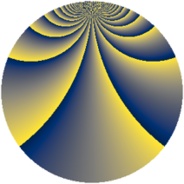# Properties

 Label 1728.2.bsLevel $1728$ Weight $2$ Character orbit 1728.bs Rep. character $\chi_{1728}(49,\cdot)$ Character field $\Q(\zeta_{36})$ Dimension $840$ Sturm bound $576$

# Related objects

## Defining parameters

 Level: $$N$$ $$=$$ $$1728 = 2^{6} \cdot 3^{3}$$ Weight: $$k$$ $$=$$ $$2$$ Character orbit: $$[\chi]$$ $$=$$ 1728.bs (of order $$36$$ and degree $$12$$) Character conductor: $$\operatorname{cond}(\chi)$$ $$=$$ $$432$$ Character field: $$\Q(\zeta_{36})$$ Sturm bound: $$576$$

## Dimensions

The following table gives the dimensions of various subspaces of $$M_{2}(1728, [\chi])$$.

Total New Old
Modular forms 3552 888 2664
Cusp forms 3360 840 2520
Eisenstein series 192 48 144

## Trace form

 $$840 q + 12 q^{3} - 12 q^{5} + O(q^{10})$$ $$840 q + 12 q^{3} - 12 q^{5} + 12 q^{11} - 12 q^{13} + 24 q^{15} - 12 q^{17} + 6 q^{19} - 12 q^{21} + 12 q^{27} - 12 q^{29} + 24 q^{31} - 24 q^{33} + 6 q^{35} - 6 q^{37} + 12 q^{43} - 12 q^{45} + 24 q^{47} - 24 q^{49} - 6 q^{51} - 24 q^{53} + 48 q^{59} - 12 q^{61} + 24 q^{63} - 24 q^{65} + 12 q^{67} - 12 q^{69} + 96 q^{75} - 12 q^{77} + 24 q^{79} - 24 q^{81} - 48 q^{83} + 18 q^{85} + 6 q^{91} - 12 q^{93} + 24 q^{95} - 24 q^{97} + 12 q^{99} + O(q^{100})$$

## Decomposition of $$S_{2}^{\mathrm{new}}(1728, [\chi])$$ into newform subspaces

The newforms in this space have not yet been added to the LMFDB.

## Decomposition of $$S_{2}^{\mathrm{old}}(1728, [\chi])$$ into lower level spaces

$$S_{2}^{\mathrm{old}}(1728, [\chi]) \cong$$ $$S_{2}^{\mathrm{new}}(432, [\chi])$$$$^{\oplus 3}$$